# OECD Social spending data analysis 4 - Calculating Confidence Interval using RUnsplashArda Demirkaynakが撮影した写真

This post is following of above post. In the previous post, I made some visualizations with R ggplot2 package. In this post. In this post I will calculate confidence intervals.

Firstly, let's see average value of variables.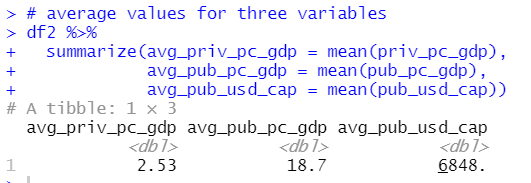I see average priv_pc_gdp is 2.53, average pub_pc_gdp is 18.7 and average pub_usd_cap is 6848.

Let's see histograms with average value.

priv_pc_gdp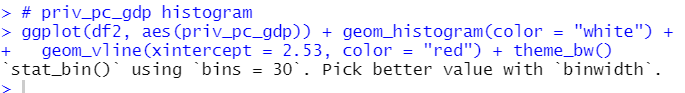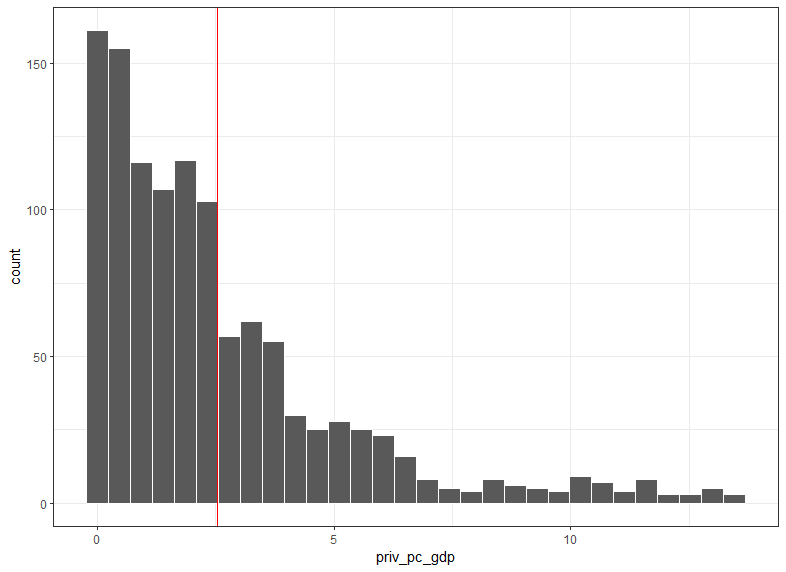pub_pc_gdp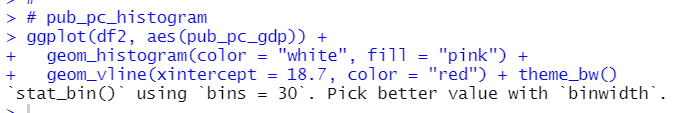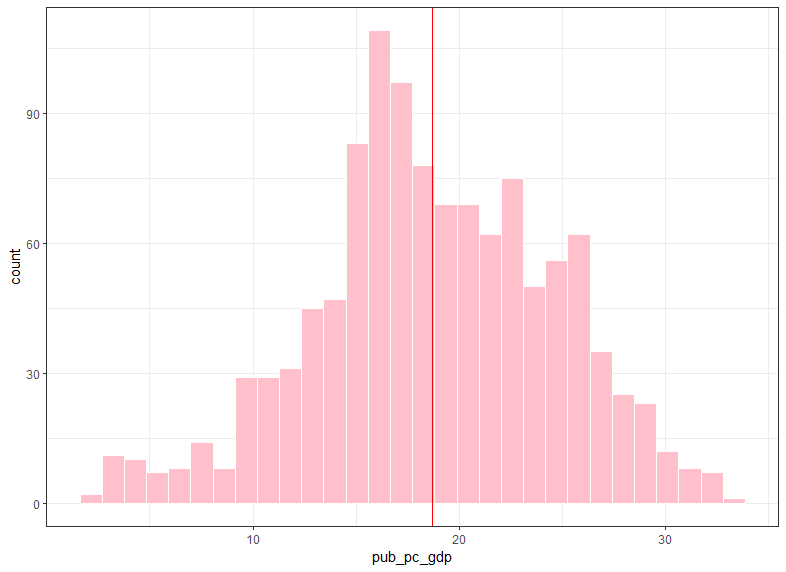pub_usd_cap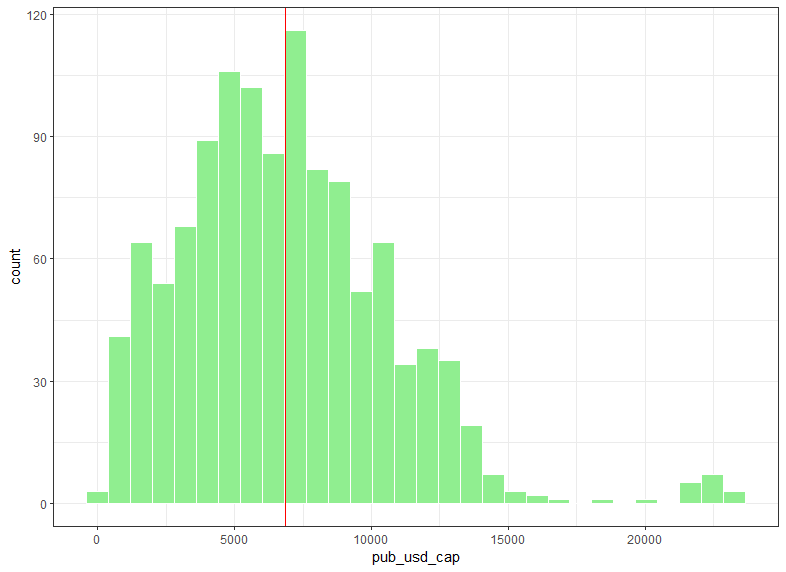I see priv_pc_gdp is the most skewed variable among those three.

To calclate confidence interval, we need standard error, which is standard deviation / sqrt(number of observations).

Let's caluclate them.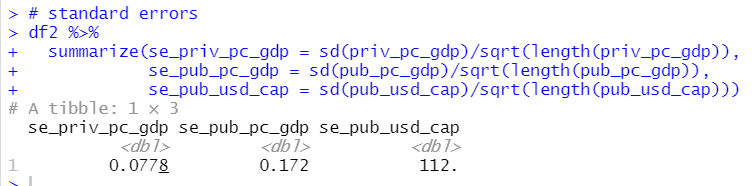So, standard error of priv_pc_gdp is 0.0778, standard error of pub_pc_gdp is 0.172 and standard error of pub_usd_cap is 112.

All right, I can calculate confidence intarval. 95% confidence interval is calculated as followings. average value -/+ 1.96*standard error.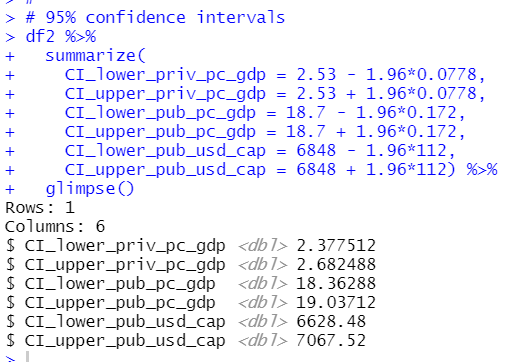So, 95% confidence interval for priv_pc_gdp is [2.378, 2.682], 95% confidence interval for pub_pc_gdp is [18.36, 10.04] and 95% confidence interval for pub_usd _cap is [6628, 7067].

Let's add confidence intervals to previous histograms.

priv_pc_gdp: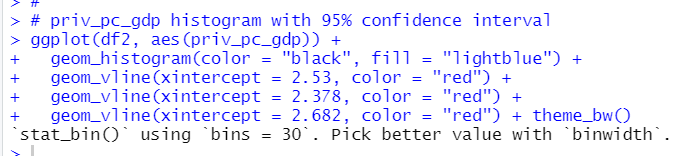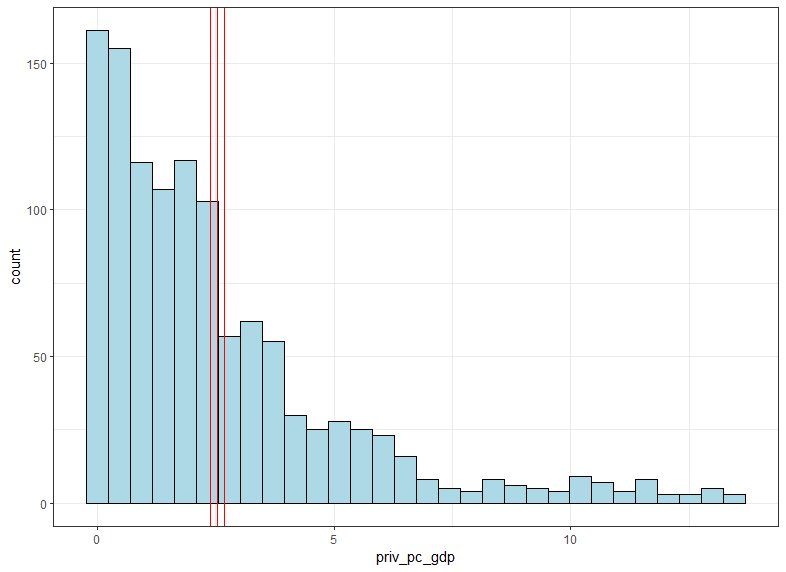pub_pc_gdp: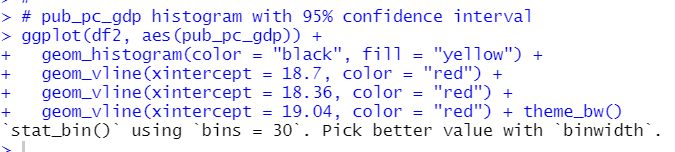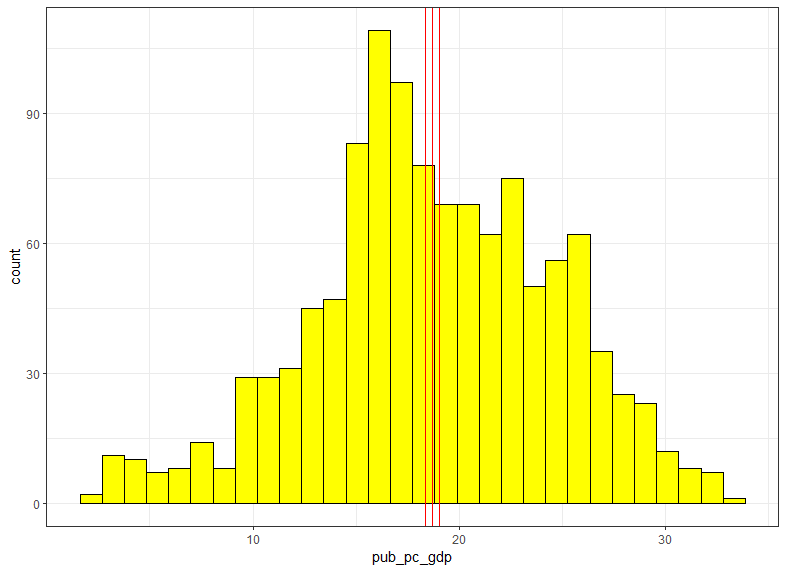pub_usd_cap: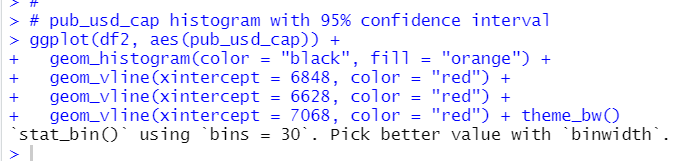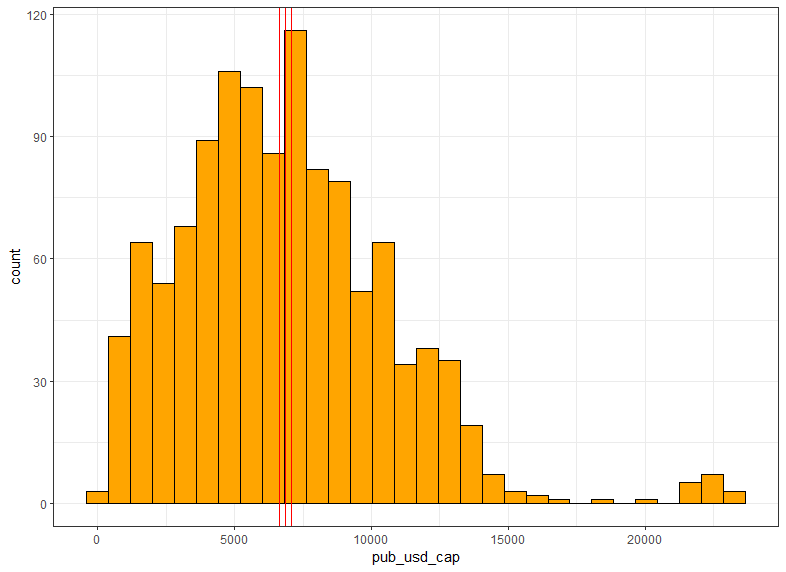That's it. Thank you!

The next post is,

To read from the 1st post,